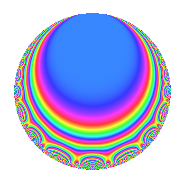# Properties

 Label 8028.2.a.iLevel 8028 Weight 2 Character orbit 8028.a Self dual Yes Analytic conductor 64.104 Analytic rank 0 Dimension 5 CM No Inner twists 1

# Related objects

## Newspace parameters

 Level: $$N$$ = $$8028 = 2^{2} \cdot 3^{2} \cdot 223$$ Weight: $$k$$ = $$2$$ Character orbit: $$[\chi]$$ = 8028.a (trivial)

## Newform invariants

 Self dual: Yes Analytic conductor: $$64.1039027427$$ Analytic rank: $$0$$ Dimension: $$5$$ Coefficient field: 5.5.1710888.1 Coefficient ring: $$\Z[a_1, \ldots, a_{7}]$$ Coefficient ring index: $$1$$ Fricke sign: $$-1$$ Sato-Tate group: $\mathrm{SU}(2)$

## $q$-expansion

Coefficients of the $$q$$-expansion are expressed in terms of a basis $$1,\beta_1,\beta_2,\beta_3,\beta_4$$ for the coefficient ring described below. We also show the integral $$q$$-expansion of the trace form.

 $$f(q)$$ $$=$$ $$q$$ $$+ ( 1 - \beta_{1} + \beta_{2} ) q^{5}$$ $$+ ( 1 - \beta_{4} ) q^{7}$$ $$+O(q^{10})$$ $$q$$ $$+ ( 1 - \beta_{1} + \beta_{2} ) q^{5}$$ $$+ ( 1 - \beta_{4} ) q^{7}$$ $$+ ( 3 + \beta_{1} - \beta_{2} - \beta_{3} - \beta_{4} ) q^{17}$$ $$+ ( -1 - \beta_{1} + \beta_{2} - \beta_{3} + \beta_{4} ) q^{19}$$ $$-2 \beta_{4} q^{23}$$ $$+ ( -2 \beta_{1} + 3 \beta_{2} - \beta_{3} ) q^{25}$$ $$+ ( -2 + 3 \beta_{1} - 2 \beta_{2} ) q^{29}$$ $$+ ( \beta_{1} - 2 \beta_{2} ) q^{31}$$ $$+ ( 2 - 2 \beta_{3} - 2 \beta_{4} ) q^{35}$$ $$+ ( 2 - \beta_{2} - \beta_{3} - \beta_{4} ) q^{37}$$ $$+ ( 3 + \beta_{4} ) q^{41}$$ $$+ ( 2 - \beta_{1} + 4 \beta_{2} + 2 \beta_{3} ) q^{43}$$ $$+ ( 4 + 2 \beta_{2} + \beta_{3} + \beta_{4} ) q^{47}$$ $$+ ( -1 - \beta_{1} + 2 \beta_{2} ) q^{49}$$ $$+ ( 1 - 3 \beta_{1} + \beta_{2} + 3 \beta_{3} + \beta_{4} ) q^{53}$$ $$+ ( 4 - 2 \beta_{1} - 2 \beta_{2} ) q^{59}$$ $$+ ( -2 - 2 \beta_{1} + 2 \beta_{3} - 2 \beta_{4} ) q^{61}$$ $$+ ( 5 - 2 \beta_{1} - \beta_{2} + \beta_{3} + 2 \beta_{4} ) q^{67}$$ $$+ ( 2 - 4 \beta_{1} + 2 \beta_{2} + 2 \beta_{4} ) q^{71}$$ $$+ ( -3 - \beta_{1} - 3 \beta_{2} + \beta_{3} ) q^{73}$$ $$+ ( 3 + 2 \beta_{1} - 3 \beta_{2} + \beta_{3} + 2 \beta_{4} ) q^{79}$$ $$+ ( 1 + 4 \beta_{1} + \beta_{2} + 2 \beta_{4} ) q^{83}$$ $$+ ( 2 - 2 \beta_{1} + 2 \beta_{2} - 2 \beta_{3} - 4 \beta_{4} ) q^{85}$$ $$+ ( 7 - 2 \beta_{1} + 2 \beta_{3} - 3 \beta_{4} ) q^{89}$$ $$+ ( 4 - 2 \beta_{1} + 4 \beta_{2} ) q^{95}$$ $$+ ( -4 + 2 \beta_{1} + 4 \beta_{2} + 2 \beta_{3} ) q^{97}$$ $$+O(q^{100})$$ $$\operatorname{Tr}(f)(q)$$ $$=$$ $$5q$$ $$\mathstrut +\mathstrut 6q^{5}$$ $$\mathstrut +\mathstrut 3q^{7}$$ $$\mathstrut +\mathstrut O(q^{10})$$ $$5q$$ $$\mathstrut +\mathstrut 6q^{5}$$ $$\mathstrut +\mathstrut 3q^{7}$$ $$\mathstrut +\mathstrut 13q^{17}$$ $$\mathstrut -\mathstrut q^{19}$$ $$\mathstrut -\mathstrut 4q^{23}$$ $$\mathstrut +\mathstrut 5q^{25}$$ $$\mathstrut -\mathstrut 11q^{29}$$ $$\mathstrut -\mathstrut 3q^{31}$$ $$\mathstrut +\mathstrut 8q^{35}$$ $$\mathstrut +\mathstrut 7q^{37}$$ $$\mathstrut +\mathstrut 17q^{41}$$ $$\mathstrut +\mathstrut 15q^{43}$$ $$\mathstrut +\mathstrut 25q^{47}$$ $$\mathstrut -\mathstrut 2q^{49}$$ $$\mathstrut +\mathstrut 3q^{53}$$ $$\mathstrut +\mathstrut 14q^{59}$$ $$\mathstrut -\mathstrut 18q^{61}$$ $$\mathstrut +\mathstrut 24q^{67}$$ $$\mathstrut +\mathstrut 14q^{71}$$ $$\mathstrut -\mathstrut 23q^{73}$$ $$\mathstrut +\mathstrut 14q^{79}$$ $$\mathstrut +\mathstrut 15q^{83}$$ $$\mathstrut +\mathstrut 6q^{85}$$ $$\mathstrut +\mathstrut 25q^{89}$$ $$\mathstrut +\mathstrut 26q^{95}$$ $$\mathstrut -\mathstrut 12q^{97}$$ $$\mathstrut +\mathstrut O(q^{100})$$

Basis of coefficient ring in terms of a root $$\nu$$ of $$x^{5}\mathstrut -\mathstrut$$ $$x^{4}\mathstrut -\mathstrut$$ $$10$$ $$x^{3}\mathstrut +\mathstrut$$ $$3$$ $$x^{2}\mathstrut +\mathstrut$$ $$12$$ $$x\mathstrut +\mathstrut$$ $$4$$:

 $$\beta_{0}$$ $$=$$ $$1$$ $$\beta_{1}$$ $$=$$ $$\nu$$ $$\beta_{2}$$ $$=$$ $$($$$$\nu^{4} - \nu^{3} - 10 \nu^{2} + 5 \nu + 10$$$$)/2$$ $$\beta_{3}$$ $$=$$ $$($$$$-3 \nu^{4} + 5 \nu^{3} + 28 \nu^{2} - 27 \nu - 26$$$$)/2$$ $$\beta_{4}$$ $$=$$ $$-2 \nu^{4} + 3 \nu^{3} + 18 \nu^{2} - 15 \nu - 13$$
 $$1$$ $$=$$ $$\beta_0$$ $$\nu$$ $$=$$ $$\beta_{1}$$ $$\nu^{2}$$ $$=$$ $$-$$$$\beta_{4}\mathstrut +\mathstrut$$ $$\beta_{3}\mathstrut -\mathstrut$$ $$\beta_{2}\mathstrut +\mathstrut$$ $$\beta_{1}\mathstrut +\mathstrut$$ $$5$$ $$\nu^{3}$$ $$=$$ $$-$$$$\beta_{4}\mathstrut +\mathstrut$$ $$2$$ $$\beta_{3}\mathstrut +\mathstrut$$ $$2$$ $$\beta_{2}\mathstrut +\mathstrut$$ $$7$$ $$\beta_{1}\mathstrut +\mathstrut$$ $$3$$ $$\nu^{4}$$ $$=$$ $$-$$$$11$$ $$\beta_{4}\mathstrut +\mathstrut$$ $$12$$ $$\beta_{3}\mathstrut -\mathstrut$$ $$6$$ $$\beta_{2}\mathstrut +\mathstrut$$ $$12$$ $$\beta_{1}\mathstrut +\mathstrut$$ $$43$$

## Embeddings

For each embedding $$\iota_m$$ of the coefficient field, the values $$\iota_m(a_n)$$ are shown below.

For more information on an embedded modular form you can click on its label.

Label $$\iota_m(\nu)$$ $$a_{2}$$ $$a_{3}$$ $$a_{4}$$ $$a_{5}$$ $$a_{6}$$ $$a_{7}$$ $$a_{8}$$ $$a_{9}$$ $$a_{10}$$
1.1
 1.42525 3.36194 −2.64396 −0.676546 −0.466688
0 0 0 −1.40326 0 −1.61825 0 0 0
1.2 0 0 0 −0.594895 0 2.48438 0 0 0
1.3 0 0 0 0.756442 0 1.69379 0 0 0
1.4 0 0 0 2.95619 0 −3.03905 0 0 0
1.5 0 0 0 4.28552 0 3.47913 0 0 0
 $$n$$: e.g. 2-40 or 990-1000 Embeddings: e.g. 1-3 or 1.5 Significant digits: Format: Complex embeddings Normalized embeddings Satake parameters Satake angles

## Inner twists

This newform does not admit any (nontrivial) inner twists.

## Atkin-Lehner signs

$$p$$ Sign
$$2$$ $$-1$$
$$3$$ $$-1$$
$$223$$ $$-1$$

## Hecke kernels

This newform can be constructed as the intersection of the kernels of the following linear operators acting on $$S_{2}^{\mathrm{new}}(\Gamma_0(8028))$$:

 $$T_{5}^{5}$$ $$\mathstrut -\mathstrut 6 T_{5}^{4}$$ $$\mathstrut +\mathstrut 3 T_{5}^{3}$$ $$\mathstrut +\mathstrut 20 T_{5}^{2}$$ $$\mathstrut -\mathstrut 4 T_{5}$$ $$\mathstrut -\mathstrut 8$$ $$T_{7}^{5}$$ $$\mathstrut -\mathstrut 3 T_{7}^{4}$$ $$\mathstrut -\mathstrut 12 T_{7}^{3}$$ $$\mathstrut +\mathstrut 35 T_{7}^{2}$$ $$\mathstrut +\mathstrut 24 T_{7}$$ $$\mathstrut -\mathstrut 72$$